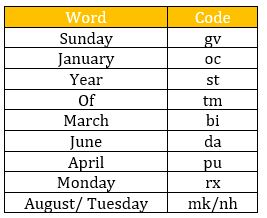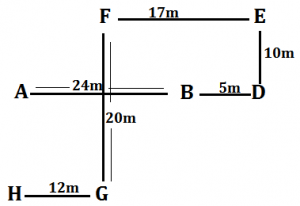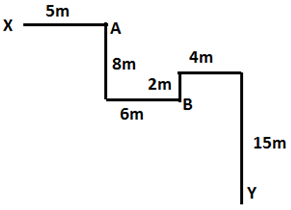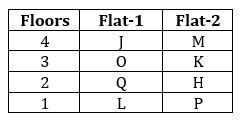Latest Banking jobs   »

# Reasoning Ability Quiz For FCI Phase I 2022- 16th December

Directions (1-5): Study the following information to answer the given questions.
In a certain code,
‘Sunday January year March’ is written as ‘bi gv oc st’
‘January April of June’ is written as ‘tm oc da pu’
‘Of Sunday August Tuesday’ is written as ‘nh mk tm gv’
‘June Monday year of’ is written as ‘da st rx tm’

Q1. In the given code language, what does the code ‘nh’ stand for?
(a) Either ‘Sunday’ or ‘year’
(b) Either ‘Tuesday’ or ‘August’
(c) Monday
(d) March
(e) of

Q2. What is the code for ‘April’ in the given code language?
(a) pu
(b) rx
(c) st
(d) da
(e) None of these

Q3. What is the code for ‘Year’ in the given code language?
(a) da
(b) gv
(c) tm
(d) rx
(e) st

Q4. What is the code for ‘Sunday of March’ in coded language?
(a) gv da tm
(b) bi oc tm
(c) pu st gv
(d) tm gv bi
(e) None of these

Q5. What may be the code for ‘August of spring year’ in coded language?
(a) nh st pu tm
(b) mk tm am st
(c) mk nh bi da
(d) tm st mk oc
(e) None of these

Directions (6-8): Study the following information carefully and answer the given questions.
Point A is 24m west of point B. Point D is 10m south of point E. Point F is 20m north of point G. Point D is 5m east of point B. point E is 17m east of F. Point H is 12m west of point G.

Q6. What is the direction of point E with respect to point A?
(a) North-west
(b) North
(c) South-west
(d) North-east
(e) None of these

Q7. What is the shortest distance between point H and point B?
(a) 15m
(b) 26m
(c) 25m
(d) 21m
(e) None of these

Q8. What is the direction of point F with respect to D?
(a) South-west
(b) North
(c) North-west
(d) East
(e) None of these

Directions (9-10): Read the following information carefully and answer the given questions.
A person starts walking from point X in east direction and walk 5m and reach at point A. From point A he takes a right turn and then a left turn and walks 8m and 6m respectively and reach at point B, from point B he goes in north direction and walks 2m and then takes two consecutive right turn and walks 4m and 15m respectively and finally reach at point Y.

Q9. What is the direction of point Y with respect to his initial point?
(a) South-east
(b) West
(c) East
(d) North-west
(e) None of these

Q10. What is the shortest distance between point A and point B?
(a) 12m
(b) 6m
(c) 10m
(d) 5m
(e) None of these

Directions (11-15): Study the following information carefully and answer the questions given below:
Eight persons are living in a building having four floors where ground floor is numbered as 1, the floor above it is numbered as 2 and so on till the topmost floor which is numbered as 4. Each floor is having two flats i.e., Flat-1 and flat-2. Flat-1 of floor-2 is exactly above the flat-1 of floor-1 and exactly below the flat-1 of floor-3 and so on. Similarly flat-2 of floor-2 is exactly above the flat-2 of floor-1 and exactly below flat-2 of floor-3 and so on. Flat-2 is in east of flat-1.
J lives to the west of M. There are two floor gaps between L and J who does not live on bottom most floor. K lives on same numbered floor as O. O lives on a floor above Q’s floor who does not live in flat-2. There is one floor gap between M and H and both live on same numbered flat. One of the persons is P who does not live in flat-1. O lives on the same numbered flat as J.

Q11. Who among the following lives on flat-1 of 2nd floor?
(a) L
(b) M
(c) Q
(d) H
(e) None of these

Q12. Who among the following lives below P’s floor?
(a) H
(b) J
(c) No one
(d) O
(e) K

Q13. Four of the following five are alike in a certain way and hence form a group, Who among the following does not belong to the group?
(a) L
(b) P
(c) Q
(d) O
(e) J

Q14. Which of the following statement/statements is/are true?
(a) J lives on the topmost floor
(b) K lives on a floor immediately above P’s floor
(c) H lives with Q on the same floor
(d) Both (a) and (c)
(e) Both (b) and (c)

Q15. Who among the following lives on the same numbered floor as P?
(a) L
(b) H
(c) M
(d) Q
(e) None of these

Solutions

Solutions (1-5):
Sol.S1. Ans. (b)
S2. Ans. (a)
S3. Ans. (e)
S4. Ans. (d)
S5. Ans. (b)

Solution (6-8):
Sol.S6. Ans. (d)
S7. Ans. (b)
S8. Ans. (c)

Solutions (9-10):
Sol.S9. Ans. (a)
S10. Ans. (c)

Solutions: (11-15):
Sol.S11. Ans. (c)
S12. Ans. (c)
S13. Ans. (b)
S14. Ans. (d)
S15. Ans. (a)#### Congratulations!Union Budget 2023-24: Free PDF